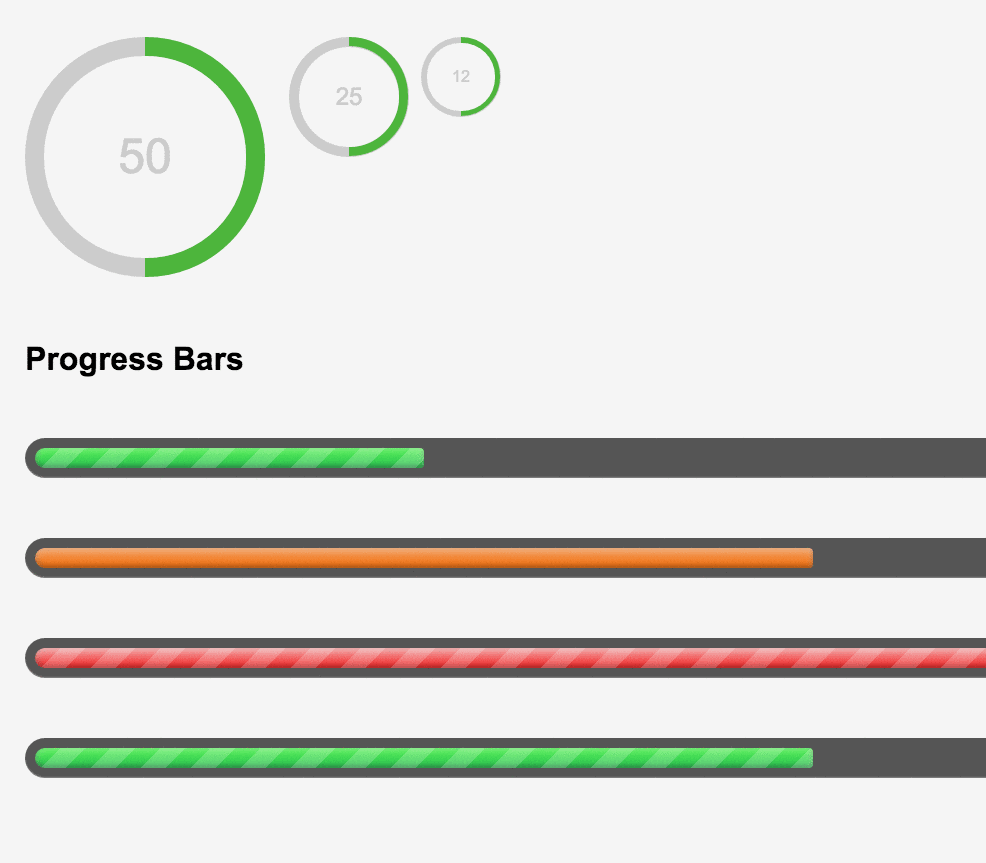### 1.circle動態使用方式

``````//首先head中引入ＣＳＳ

//class解析c100=灰色100%bar條 ; big=大圓（可更換small）會變小圓;green=顏色（可依個人喜好自行增加）
50//此ＩＤ是為了jquery控制，當抓到裡面的值多少時，會有多長的bar

//控制bar長度的地方

//引入jquery.js

jQuery(function() {
var x = \$('#test').html();//宣告一個變數x＝test裡面的內容（上方html裡面的內容）

});

// 數字動態計數效果js，寫入即可
\$(function() {
function count(\$this){
var current = parseInt(\$this.html(), 10);
\$this.html(++current);
if(current !== \$this.data('count')){
setTimeout(function(){count(\$this)}, 50);
}
}
\$("#test").each(function() {   //此ＩＤ="test"可依你的div下的id做修改
\$(this).data('count', parseInt(\$(this).html(), 10));
\$(this).html('0');
count(\$(this));
});
});

``````

### 2.progress Bars動態使用方式

``````//首先head中引入ＣＳＳ

//引入jquery.js

//progress bar
\$(function() {    //帶入此段code會依span style="width:25%" 的width寬度來決定bar的寬度
\$(".meter > span").each(function() {
\$(this)
.data("origWidth", \$(this).width())
.width(0)
.animate({
width: \$(this).data("origWidth")
}, 1200);
});
});

``````